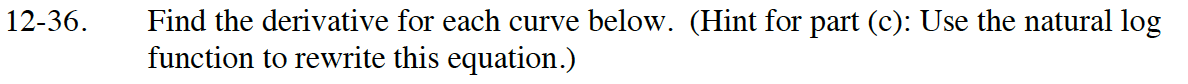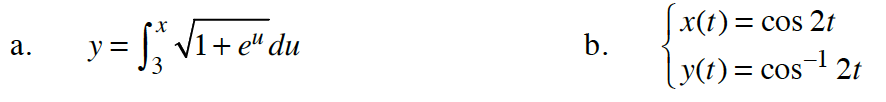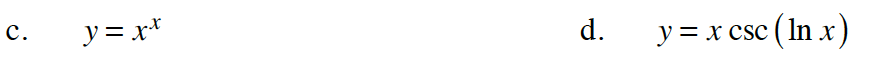### Home > CALC > Chapter Ch12 > Lesson 12.1.3 > Problem12-36

12-36.$\frac{d}{dx}y=\frac{d}{dx}\int_3^x\sqrt{1+e^u}du$

$\frac{dy}{dx}=\sqrt{1+e^x}$

$\frac{dy}{dx}=\frac{y^\prime(t)}{x^\prime(t)}$$\ln(y)=x\ln(x)$

$\frac{1}{y}y^\prime=\ln(x)+1$

$y^\prime=y(\ln(x)+1)$

$y^\prime=x^x(\ln(x)+1)$

$y^\prime=\csc(\ln(x))+x(-\csc(\ln(x))\cot(\ln(x))\cdot\frac{1}{x}$